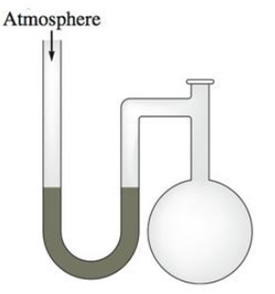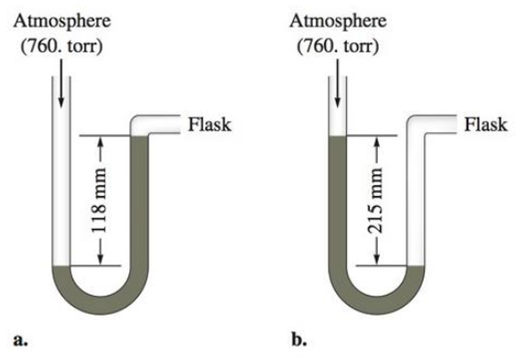# A diagram for an open-tube manometer is shown below. If the flask is open to the atmosphere, the mercury levels are equal. For each of the following situations where a gas is contained in the flask, calculate the pressure in the flask in torr, atmospheres, and pascals. c. Calculate the pressures in the flask in parts a and b (in torr) if the atmospheric pressure is 635 torr.### Chemistry: An Atoms First Approach

2nd Edition
Steven S. Zumdahl + 1 other
Publisher: Cengage Learning
ISBN: 9781305079243

#### Solutions

Chapter
Section### Chemistry: An Atoms First Approach

2nd Edition
Steven S. Zumdahl + 1 other
Publisher: Cengage Learning
ISBN: 9781305079243
Chapter 8, Problem 41E
Textbook Problem
1925 views

## A diagram for an open-tube manometer is shown below.If the flask is open to the atmosphere, the mercury levels are equal. For each of the following situations where a gas is contained in the flask, calculate the pressure in the flask in torr, atmospheres, and pascals.c. Calculate the pressures in the flask in parts a and b (in torr) if the atmospheric pressure is 635 torr.

(a)

Interpretation Introduction

Interpretation:

The pressure of the gases in given two situations of manometers (a) and (b) should be determined in units of torr, atm and pascals when the manometer shows a reading of 118mm and 215mm respectively. And also calculate the pressure of the gases in given two situations of manometers (a) and (b) If the atmospheric pressure is 635 torr.

Concept Introduction:

The manometer is a devise used measure the pressure of a gas. The pressure of gas is determined by the value of ‘h’ shown by the manometer. This ‘h’-value is added or subtracted with atmospheric pressure to determine the pressure of gas.

If the flask side mercury level is decreased after the filling of gas, then the ‘h’-value will be added to atmospheric pressure to get the pressure of gas.

If the flask side mercury level is increased after the filling of gas, then the ‘h’-value will be subtracted from the atmospheric pressure to get the pressure of gas.

The pressure equivalent of ‘h’ value is,

h=1mm=1mmHg

Pressure of a substance can be stated in various units. The units of pressure are interconvertible. The relations between units of pressure are,

• Since the unit mm Hg and the unit torr is used interchangeably.

1mmHg = 1torr

• Conversion of 1 torr into atm is,

1mmHg = 1760atm

• The 1 mm Hg pressure in Pa unit is,

1mmHg = 101325760Pa

### Explanation of Solution

The given ‘h’ value for the gas in manometer is 118mm. The picture of manometer shows the    flask side mercury level is increased after the filling of gas.

Hence the equation for finding the pressure of gas is,

Atmospheric pressureh(inmm)

That is,

=    (760118)mmHg

=    642mmHg

The calculated pressure is 642 mm Hg; the mm Hg and torr units are used interchangeably,

Therefore,

642mmHg = 642torr

The calculated pressure is 642mm Hg. So the pressure in atm unit is,

642mmHg = 642mm</

(b)

Interpretation Introduction

Interpretation:

The pressure of the gases in given two situations of manometers (a) and (b) should be determined in units of torr, atm and pascals when the manometer shows a reading of 118mm and 215mm respectively. And also calculate the pressure of the gases in given two situations of manometers (a) and (b) If the atmospheric pressure is 635 torr.

Concept Introduction:

The manometer is a devise used measure the pressure of a gas. The pressure of gas is determined by the value of ‘h’ shown by the manometer. This ‘h’-value is added or subtracted with atmospheric pressure to determine the pressure of gas.

If the flask side mercury level is decreased after the filling of gas, then the ‘h’-value will be added to atmospheric pressure to get the pressure of gas.

If the flask side mercury level is increased after the filling of gas, then the ‘h’-value will be subtracted from the atmospheric pressure to get the pressure of gas.

The pressure equivalent of ‘h’ value is,

h=1mm=1mmHg

Pressure of a substance can be stated in various units. The units of pressure are interconvertible. The relations between units of pressure are,

• Since the unit mm Hg and the unit torr is used interchangeably.

1mmHg = 1torr

• Conversion of 1 torr into atm is,

1mmHg = 1760atm

• The 1 mm Hg pressure in Pa unit is,

1mmHg = 101325760Pa

(c)

Interpretation Introduction

Interpretation:

The pressure of the gases in given two situations of manometers (a) and (b) should be determined in units of torr, atm and pascals when the manometer shows a reading of 118mm and 215mm respectively. And also calculate the pressure of the gases in given two situations of manometers (a) and (b) If the atmospheric pressure is 635 torr.

Concept Introduction:

The manometer is a devise used measure the pressure of a gas. The pressure of gas is determined by the value of ‘h’ shown by the manometer. This ‘h’-value is added or subtracted with atmospheric pressure to determine the pressure of gas.

If the flask side mercury level is decreased after the filling of gas, then the ‘h’-value will be added to atmospheric pressure to get the pressure of gas.

If the flask side mercury level is increased after the filling of gas, then the ‘h’-value will be subtracted from the atmospheric pressure to get the pressure of gas.

The pressure equivalent of ‘h’ value is,

h=1mm=1mmHg

Pressure of a substance can be stated in various units. The units of pressure are interconvertible. The relations between units of pressure are,

• Since the unit mm Hg and the unit torr is used interchangeably.

1mmHg = 1torr

• Conversion of 1 torr into atm is,

1mmHg = 1760atm

• The 1 mm Hg pressure in Pa unit is,

1mmHg = 101325760Pa

### Still sussing out bartleby?

Check out a sample textbook solution.

See a sample solution

#### The Solution to Your Study Problems

Bartleby provides explanations to thousands of textbook problems written by our experts, many with advanced degrees!

Get Started

Find more solutions based on key concepts
Which of these advantages do sports drinks provide over plain water? They taste good and so may lead people to ...

Nutrition: Concepts and Controversies - Standalone book (MindTap Course List)

Describe what is meant by the term primary structure of proteins.

Chemistry for Today: General, Organic, and Biochemistry

An outfielder throws a baseball to his catcher in an attempt to throw out a runner at home plate. The ball boun...

Physics for Scientists and Engineers, Technology Update (No access codes included)

How is bilateral symmetry different from radial symmetry?

Oceanography: An Invitation To Marine Science, Loose-leaf Versin